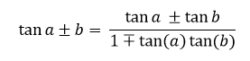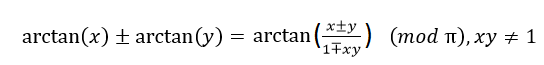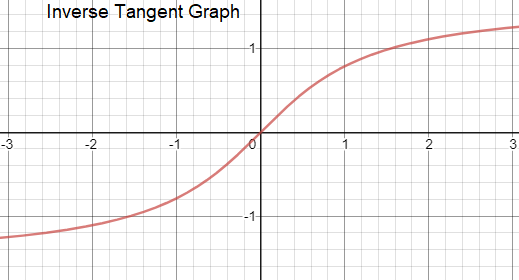# Inverse Tan

Inverse tan is the inverse function of the trigonometric function ‘tangent’. It is used to calculate the angle by applying the tangent ratio of the angle, which is the opposite side divided by the adjacent side of the right triangle. Based on this function, the value of tan 1 or arctan 1 or tan 10, etc. can be determined. It is naming convention for all inverse trigonometric functions to use the prefix ‘arc’ and hence inverse tangent is denoted by arctan. Although it is not uncommon to use tan-1, we will use arctan throughout this article.

## Inverse Tangent Formula

The formula for adding two inverse tangent function is derived from tan addition formula.In this formula, by putting a = arctan x and b = arctan y, we getFor Integration:

Some of the important formulae for calculating integrals of expressions involving the arctan function are:

∫ arctan (x)dx = {x arctan (x)} – {In (x2+1)/2} + C

∫ arctan (ax)dx = {x arctan (ax)}- {In (a2x2+1)/2a} + C

∫ x arctan (ax)dx = {x2 arctan (ax)/2} – {arctan(ax)/2a2 } – {x / 2a} + C

∫ x2 arctan (ax)dx = {x3 arctan (ax)/3} – {In (a2x+ 1)/6a3 } – {x2/6a} + C

## Calculus of Arctan Function

This section gives the formula to calculate the derivative and integral of the arctan function.

Derivative of tan inverse x

The differentiation of tan inverse x is given below. The derivative of arctan x is denoted by d/dx(arctan(x)) and for complex values of x , the derivative is equal to 1/1+x2 for x ≠ -i, +i.

Therefore, the differentiation of tan inverse x is given by:

 $$\begin{array}{l}\frac{d}{dx}tan^{-1}x = \frac{1}{1 + x^2}\end{array}$$

Integral of inverse tan

For obtaining an expression for the definite integral of the inverse tan function, the derivative is integrated and the value at one point is fixed. The expression is:

$$\begin{array}{l}arctan (x) = \int_{0}^{x}\frac{1}{y^{2}+1}dy\end{array}$$

## Inverse Tan Graph### Relationship Between Inverse Tangent Function and Other Trigonometric Functions

Consider a triangle whose length of adjacent and opposite are 1 and x respectively. Therefore, the length of the hypotenuse is √(1+x2). For this triangle, if the angle θ is the arctan, then the following relationships hold true for the three basic trigonometric functions:

$$\begin{array}{l}Sin (arctan(x)) = \frac{x}{\sqrt{1+x^{2}}}\end{array}$$
$$\begin{array}{l}Cos (arctan(x)) =\frac{1}{\sqrt{1+x^{2}}}\end{array}$$

Tan (arctan(x)) = x

## Inverse Tangent Properties

The basic properties of the inverse tan function (arctan) are listed below:

Notation: y = arctan (x)

Defined as: x = tan (y)

Domain of the ratio: all real numbers

Range of the principal value in radians: -π/2 < y < π/2

Range of the principal value in degrees: -90° < y < 90°

### What is the Value of tan-1 Infinity?

To calculate the value of the tan inverse of infinity(∞), we have to check the trigonometry table. From the table we know, the tangent of angle π/2 or 90° is equal to infinity, i.e.,

tan 90° = ∞ or tan π/2 = ∞

Therefore, tan-1 (∞) = π/2 or tan-1 (∞) = 90°

## Solved Examples On Inverse Tan

Example 1:

$$\begin{array}{l}\text{Prove that }4\left(2 \tan ^{-1} \frac{1}{3}+\tan ^{-1} \frac{1}{7}\right)=\pi\end{array}$$

Solution:

$$\begin{array}{l}\text{Consider }2 \tan ^{-1} \frac{1}{3} \end{array}$$

This can also be written as:

$$\begin{array}{l}tan ^{-1} \frac{1}{3}+tan ^{-1} \frac{1}{3}\\=tan ^{-1}\left(\frac{\frac{1}{3}+\frac{1}{3}}{1-\frac{1}{3} . \frac{1}{3}}\right) \\ =tan ^{-1} \frac{3}{4}\end{array}$$

$$\begin{array}{l}\text{Now, LHS = }4\left(2 tan ^{-1} \frac{1}{3}+tan ^{-1} \frac{1}{7}\right) \\ =4\left(tan ^{-1} \frac{3}{4}+tan ^{-1} \frac{1}{7}\right) \\ =4\ tan ^{-1}\left(\frac{\frac{3}{4}+\frac{1}{7}}{1-\frac{3}{4} . \frac{1}{7}}\right) \\ =4\ tan ^{-1}\left(\frac{25}{28} \times \frac{28}{25}\right) \\ =4\ tan ^{-1} 1 \\ =4 \times \frac{\pi}{4} \\ =\pi\end{array}$$

= RHS

Hence proved.

Example 2:

$$\begin{array}{l}\text{Show that }\tan ^{-1} \frac{1}{4}+\tan ^{-1} \frac{2}{9}+\tan ^{-1} \frac{1}{5}+\tan ^{-1} \frac{1}{8}=\pi / 4\end{array}$$

Solution:

LHS =

$$\begin{array}{l}\begin{array}{l} =tan ^{-1} \frac{1}{4}+tan ^{-1} \frac{2}{9}+tan ^{-1} \frac{1}{5}+tan ^{-1} \frac{1}{8} \\ =tan ^{-1} \frac{\frac{1}{4}+\frac{2}{9}}{1-\frac{1}{4} . \frac{2}{9}}+tan ^{-1} \frac{\frac{1}{5}+\frac{1}{8}}{1-\frac{1}{5} .\frac{1}{8}} \\ =tan ^{-1}\left(\frac{17}{36} \times \frac{36}{34}\right)+tan ^{-1}\left(\frac{13}{40} \times \frac{40}{39}\right) \\ =tan ^{-1} \frac{1}{2}+tan ^{-1} \frac{1}{3} \\ =tan ^{-1} \left ( \frac{\frac{1}{2}+\frac{1}{3}}{1-\frac{1}{2} .\frac{1}{3}} \right ) \\ =tan ^{-1}(1) \\ =\frac{\pi}{4} \end{array}\end{array}$$

= RHS

Example 3:

$$\begin{array}{l}\text{Find the value of x, for which }3 \tan ^{-1} \frac{1} {2+\sqrt{3}}-\tan ^{-1}\frac{1}{x}=\tan ^{-1}\frac{1}{3}\end{array}$$

Solution:

Consider, tan 15° = tan(45° – 30°)

= (tan 45° – tan 30°)/(1 – tan 45° tan 30°)

$$\begin{array}{l}\begin{array}{l} \Rightarrow \tan 15^{\circ}=\frac{1-\frac{1}{\sqrt{3}}}{1+\frac{1}{\sqrt{3}}} \\ \Rightarrow \tan 15^{\circ}=\frac{\sqrt{3}-1}{\sqrt{3}+1} \\ \Rightarrow \tan 15^{\circ}=\frac{(\sqrt{3}-1)(\sqrt{3}+1)}{(\sqrt{3}+1)(\sqrt{3}+1)} \\ \Rightarrow \tan 15^{\circ}=\frac{3-1}{4+2 \sqrt{3}} \\ \Rightarrow \tan 15^{\circ}=\frac{1}{2+\sqrt{3}} \\ \Rightarrow \tan ^{-1}\left(\frac{1}{2+\sqrt{3}}\right)=15^{\circ} \\ \Rightarrow \tan ^{-1}\left(\frac{1}{2+\sqrt{3}}\right)=\frac{\pi}{12}\end{array}\end{array}$$

From the given,

$$\begin{array}{l}\begin{array}{l}3 \tan ^{-1} \frac{1}{2+\sqrt{3}}-\tan ^{-1} \frac{1}{x}=\tan ^{-1} \frac{1}{3} \\ \Rightarrow 3 \cdot \frac{\pi}{12}-\tan ^{-1} \frac{1}{x}=\tan ^{-1} \frac{1}{3} \\ \Rightarrow-\tan ^{-1} \frac{1}{x}=\tan ^{-1} \frac{1}{3}-\frac{\pi}{4} \\ \Rightarrow \tan ^{-1} \frac{1}{x}=\tan ^{-1} 1-\tan ^{-1} \frac{1}{3}\ [ since, \frac{\pi}{4}=\tan ^{-1} 1] \\ \Rightarrow \tan ^{-1} \frac{1}{x}=\tan ^{-1} \frac{1-\frac{1}{3}}{1+1 . \frac{1}{3}} \end{array}\end{array}$$

⇒ tan-1(1/x) = tan-1(1/2)

⇒ 1/x = 1/2

⇒ x = 2

Therefore, the value of x is 2.

### Practice Problems

1. $$\begin{array}{l}\text{Show that }4 tan ^{-1} \frac{1}{5}-tan ^{-1} \frac{1}{70}+tan ^{-1} \frac{1}{99}=\frac{\pi}{4}\end{array}$$
2. If y = tan-1(sec x + tan x), then find dy/dx.
3. Find the value of x if tan-1[(x – 1)/(x – 2)] + tan-1[(x + 1)/(x + 2)] = π/4.